# Linear Interpolation for Excel

Click here to go to our page on using linear interpolation to solve "one-way" equations

This page has one of our highest page views rates, because it is useful well beyond the microwave engineering community.  Here's a quote from Josh who sent us an email in Febrary 2015:

"I just wanted to drop a quick note that your advice about using Index and Match Functions just helped me finish a project.  I couldn't find this anywhere else and it was fantastic help!"

There are many times in engineering where you find yourself interpolating between data points. In microwave engineering, this happens in power bench measurements all the time (you only get a limited set of calibration data with a power head). Also when you have manufacturer's S-parameters, they are not always at the exact frequency you want.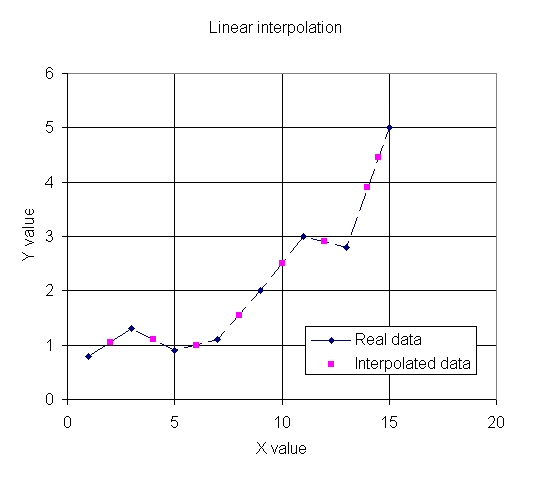Before we even get into the obtuse EXCEL calculation, let's point out that there is no excuse for Microsoft Excel not to have this function built in. MathCAD has it (and tons more cool functions!), MathCAD calls it the LINTERP function. BTW, what do you call the function when your kid wants help with their math homework? That's MathDAD!

Interpolation means fitting Y-value data to to an X-value that is somewhere between two data points, using a straight line. Simple in concept, a royal pain to do in a spreadsheet.

Note 1: fitting a "spline" to data is often much more accurate than linear interpolation.  We don't discuss that here, but you can get spline data out of Excel.

Note 2: when interpolating S-parameter data, is recommended that you interpolate magnitude and angle (you could call this "radial" interpolation) rather than real and imaginary data.

#### Interpolation or extrapolation?

Interpolation is "faking" data points that are contained inside a wide dataset. Extrapolation is faking data points outside the dataset. Extrapolation is wishful thinking, and can get you in trouble in microwave engineering. Try to see what extrapolating the response of a bandpass filter does outside the passband sometime if you need proof!

### What is interpolation?

Any high school graduate should be able to tell you the formula for linear interpolation. Especially after they look it up on Wikipedia! Below is a close-up of an interpolated data point. X1 and Y1 are "real" data points, so are X2 and Y2. We seek to find the Y value for an arbitrary X value between these two points, such that it is on the straight line that is drawn between them.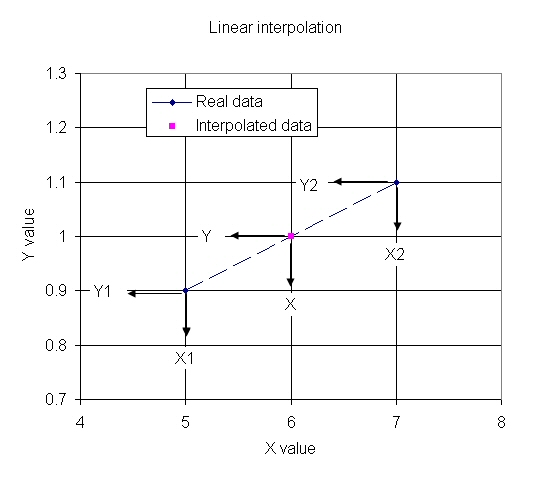This is the linear interpolation function:

Y=Y1+(X-X1)x(Y2-Y1)/(X2-X1)

Simple, n’est-ce pas?

Interpolation is used every day in engineering, linear is just one method. Other more complicated methods include fitting a cubic spline to a data set (which is what Excel does when it draws curved lines in a graph) or logarithmic interpolation, and even log-linear interpolation (linear on one axis, logarithmic on the other). Actually, if you interpolate S-parameter magnitudes in dB over frequency, you are doing log-lin interpolation.

By the way, our spreadsheet can interpolate on log-log and log-lin axes. All you have to do is convert your data to log format first, then interpolate, then "unconvert" from log.

### Creating the function in Excel

In Excel, creating the interpolation function uses a mix of other functions INDEX and MATCH functions (there may be other ways to do it but this is how we did it). You have to find the next lower value and the next higher value in the array for X and Y. MATCH is used to find X1 and X2, while INDEX returns the Y1 and Y2 values that MATCH points to.

The formula for interpolating a Y value for a X value contained in cell C10 is given below. In this case the data set is contained in rows 10 to 17 (column A is X data, column B is Y data), and it is in ascending order.

=INDEX(\$A\$10:\$B\$17, MATCH(C10,\$A\$10:\$A\$17,1),2)+
(C10-INDEX(\$A\$10:\$B\$17, MATCH(C10,\$A\$10:\$A\$17,1),1))*
(INDEX(\$A\$10:\$B\$17, MATCH(\$C10,\$A\$10:\$A\$17,1)+1,2)-
INDEX(\$A\$10:\$B\$17, MATCH(\$C10,\$A\$10:\$A\$17,1),2))/
(INDEX(\$A\$10:\$B\$17, MATCH(\$C10,\$A\$10:\$A\$17,1)+1,1)-
INDEX(\$A\$10:\$B\$17, MATCH(\$C10,\$A\$10:\$A\$17,1),1))

Yikes!

MATCH(C10,\$A\$10:\$A\$17,1) finds the largest value that is less than or equal to "lookup_value", which in this case is the X data. The is "X1" in the graph.

MATCH(\$C10,\$A\$10:\$A\$17,1)+1,2) finds the next value that is just a little bigger than X1, this is X2 in the graph.

Match is used to find the Y1 and Y2 values.

Than it's just a matter of computation:

Y=Y1+(X-X0)*(Y2-Y1)/(X2-X1)

Here's a message board post on another web site that also attempts to describe the solution. We think we offer a better explanation, but feel free to comment if you see any points that need clarification.

Now it's time for two examples.

### Noise source example

A noise source is used in noise figure measurements. It provides two noise states, the excess noise ratio is the difference between them, usually expressed in dB. There is a limited set of calibration data, and it is usually attached to the noise source by the factory, like the photo of Agilent 346B noise source below (actually this is an old "HP" unit, newer units are branded as "Agilent").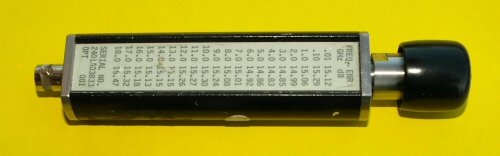We entered the data into the interpolation spreadsheet, and interpolated values between the calibrated points, shown below. Notice how interpolation tends to smooth data!

.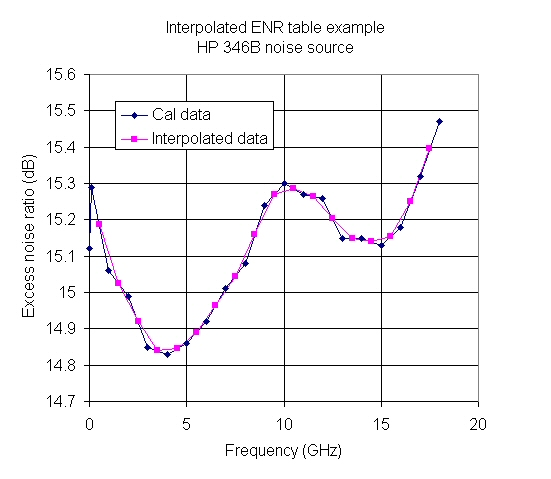Here's an 8485A power head from Agilent, used in power meter measurements. It has calibration data from 2 to 26.5 GHz. Actually the "reference cal factor" is another data point at 50 MHz, in this case it is 99%.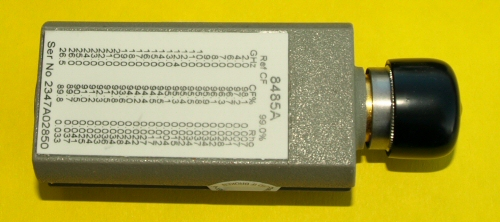We entered the data into the spreadsheet, and interpolated points between calibration data as shown below.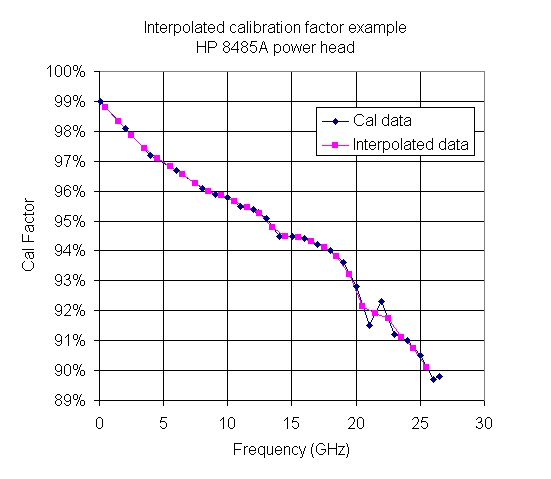### S-parameter example

S-parameters can be interpolated over frequency, but there's two issues to consider. Should you interpolate the magnitude in linear units, in dB, or should you convert magnitude/phase to real/imaginary and interpolate that? In the case of Agilent's Advanced Design System, you'd use the "native units", so you'd just interpolate whatever the data came in as.

The other issue you have to deal with is when you interpolate phase data between wo adjacent data points where the phase angle wraps from -180 to +180 degrees; you can end up on the opposite side of the Smith chart.

Below is a plot of interpolated S-parameter angle data that we created from an Hittite HMC548LP3 that we downloaded from the Hittite web site. The frequencies are incremented every 11.962 MHz, which is a result of measuring the device from 500 to 3000 MHz in 210 steps. If you look closely there is one errant point in the interpolated S21 angle, this happened at 1500 MHz because the phase of the two adjacent data points wrapped past 180 degrees. One of these days we'll put in a fix for that!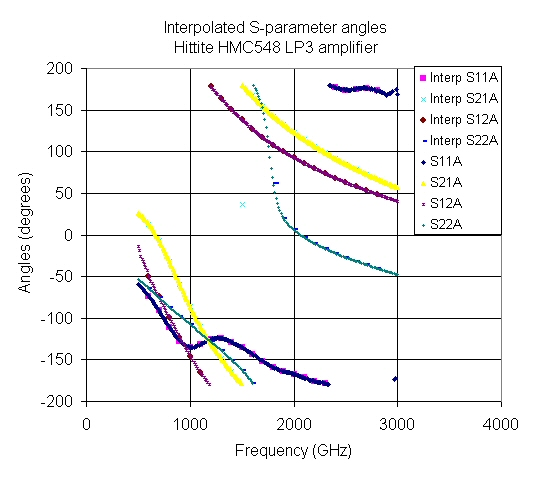Author : Unknown Editor